Previous: 3.1.1 One-Dimensional Point Response Up: 3.1 Analytical Method Next: 3.1.3 Three-Dimensional Point Response

## 3.1.2 Two-Dimensional Point Response Functions

Two-dimensional point response functions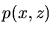are modeled by combining two of the functions presented in Sec. 3.1.1. One vertical function is multiplied with a lateral function, which additionally meets the requirement that its projected range is zero. Furthermore the moments of the lateral function depend on the vertical coordinate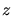. Worth mentioning is that a coordinate system is used whereis parallel to the ion beam direction.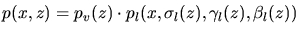(3.40)

Usually it is also assumed that the lateral function is a symmetric function which requires besides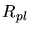also the lateral skewnessto be zero. Therefore mainly Gaussian, Pearson II and Person VII functions are used. Besides a so called modified Gaussian function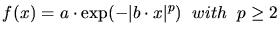(3.41)

is sometimes applied for the case that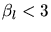. There is an explicit relation between the kurtosis and the power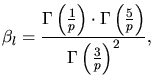(3.42)

whereis the Gamma function.

, , ,  provide calibrated two-dimensional point response functions for the implantation with boron, phosphorus, arsenic and antimony ions into amorphous and crystalline silicon, into silicon dioxide and silicon nitride.

A. Hoessiger: Simulation of Ion Implantation for ULSI Technology